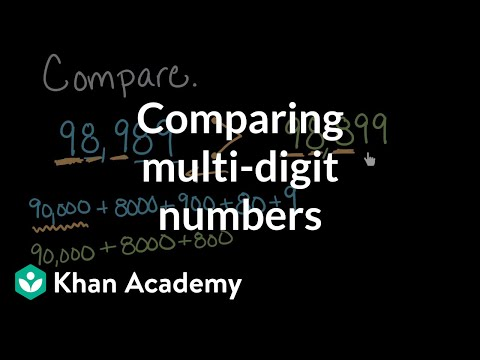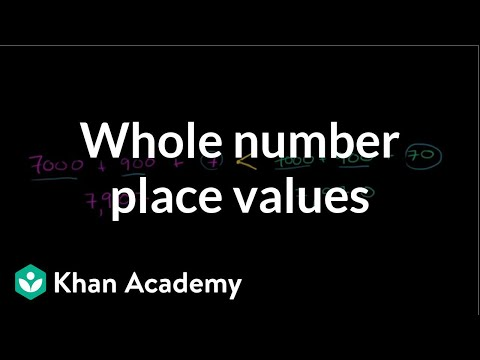Video

# Place value when multiplying and dividing by 10 (Full video)

Khan Academy

## Supporting grades: 4

Description: Lindsay discusses how multiplying and dividing by 10 affects place value. Because multiplying by 10 has some patterns in math that we can use to help us solve. One pattern we can think of when we multiply by 10 is if we take a whole number and multiply it by 10, we'll simply add a zero to the end of our whole number. So, for example, if we have a whole number like nine, and we multiply by 10, our solution will be a nine with one zero at the end.

You must log inorsign upif you want to:*

*Teacher Advisor is 100% free.

### Other videos you might be interested in### Comparing multi-digit numbers (Full video)

#### Khan Academy